Question

# Two identical point charges (q = +8.10 x 10-6 C) are fixed at opposite corners of...

Two identical point charges (q = +8.10 x 10-6 C) are fixed at opposite corners of a square whose sides have a length of 0.460 m. A test charge (q0 = -3.30 x 10-8 C), with a mass of 6.60 x 10-8 kg, is released from rest at one of the corners of the square. Determine the speed of the test charge when it reaches the center of the square.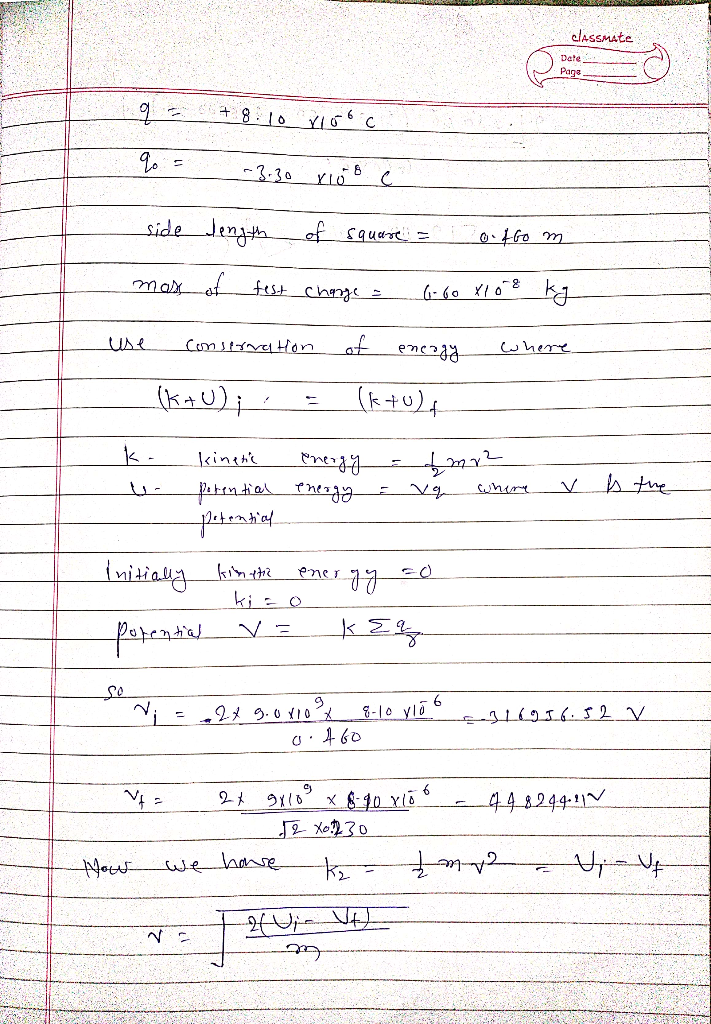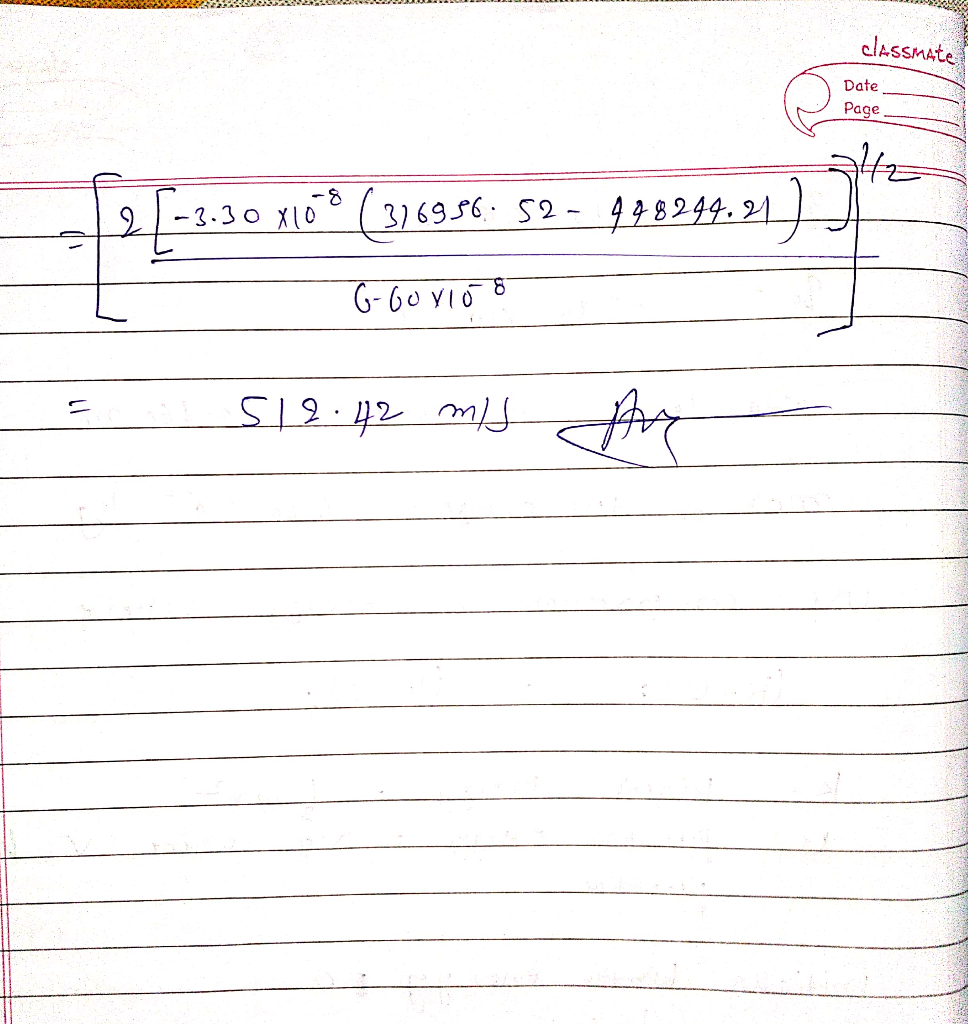#### Earn Coins

Coins can be redeemed for fabulous gifts.

Similar Homework Help Questions
• ### Two identical point charges (q = +7.60 x 10-6 C) are fixed at opposite corners of...

Two identical point charges (q = +7.60 x 10-6 C) are fixed at opposite corners of a square whose sides have a length of 0.670 m. A test charge (q0 = -7.50 x 10-8 C), with a mass of 6.50 x 10-8 kg, is released from rest at one of the corners of the square. Determine the speed of the test charge when it reaches the center of the square.

• ### Two identical point charges (q = +8.40 x 10-6 C) are fixed at opposite corners of...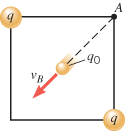Two identical point charges (q = +8.40 x 10-6 C) are fixed at opposite corners of a square whose sides have a length of 0.640 m. A test charge (q0 = -2.80 x 10-8 C), with a mass of 7.20 x 10-8 kg, is released from rest at one of the corners of the square. Determine the speed of the test charge when it reaches the center of the square.

• ### Two identical point charges (q = +2.90 x 10-6 C) are fixed at opposite corners of...

Two identical point charges (q = +2.90 x 10-6 C) are fixed at opposite corners of a square whose sides have a length of 0.540 m. A test charge (q0 = -1.80 x 10-8 C), with a mass of 9.30 x 10-8 kg, is released from rest at one of the corners of the square. Determine the speed of the test charge when it reaches the center of the square.

• ### Two identical point charges (q = +2.50 x 10-6 C) are fixed at opposite corners of...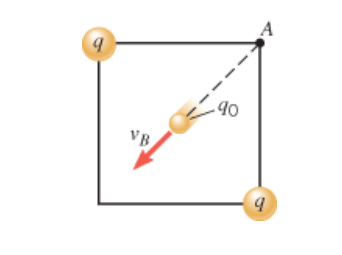Two identical point charges (q = +2.50 x 10-6 C) are fixed at opposite corners of a square whose sides have a length of 0.420 m. A test charge (q0 = -7.10 x 10-8 C), with a mass of 5.70 x 10-8 kg, is released from rest at one of the corners of the square. Determine the speed of the test charge when it reaches the center of the square. 2 40 go

• ### Two identical point charges (q = +9.60 x 10-6 C) are fixed at opposite corners of...

Two identical point charges (q = +9.60 x 10-6 C) are fixed at opposite corners of a square whose sides have a length of 0.580 m. A test charge (q0 = -7.90 x 10-8 C), with a mass of 1.70 x 10-8 kg, is released from rest at one of the corners of the square. Determine the speed of the test charge when it reaches the center of the square. Please include units. Thank you much. http://tinypic.com/m/k144tv/1

• ### Identical point charges

Two identical point charges (q = +6.95 10-6 C) are fixed at diagonally opposite corners of a square with sides of length 0.525 m. A test charge (q0 = -2.65 10-8 C),with a mass of 7.15 10-8 kg, is released from rest at one of the empty corners of the square. Determine the speed of the test charge when it reaches the center of thesquare.----------- m/s

• ### Chapter 19, Problem 13 Two point charges, +3.10 HC and -6.60 uC, are separated by 2.80...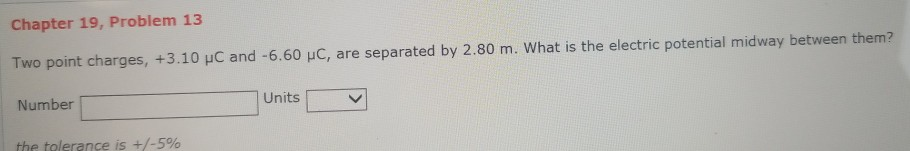Chapter 19, Problem 13 Two point charges, +3.10 HC and -6.60 uC, are separated by 2.80 m. What is the electric potential midway between them? Number the tolerance is +/-5% Units Chapter 19, Problem 24 Two identical point charges (q-+1.30 x 10。C) are fixed at opposite corners of a square whose sides have a length of 0.540 m. A test charge (a)- 9.60 x 10 C 1.40 x 10 kg, is released from rest at one of the corners of...

• ### Identical point charges q-15.00μC are placed at opposite corners of a square. The length of each...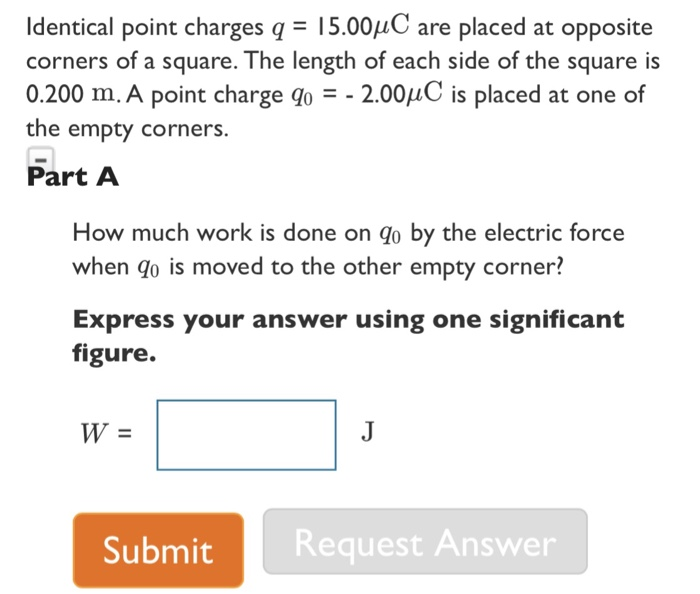Identical point charges q-15.00μC are placed at opposite corners of a square. The length of each side of the square is 0.200 m. A point charge q0--200μC is placed at one of the empty corners. Part A How much work is done on qo by the electric force when go is moved to the other empty corner? Express your answer using one significant figure. Submit Request Answer

• ### In the figure, the black dots are four identical point charges, situated at the corners of...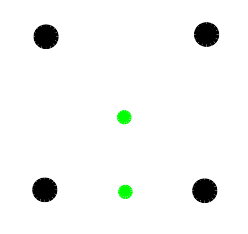In the figure, the black dots are four identical point charges, situated at the corners of a perfect square. The charges are 8 microCoulombs each and the square is 5 meters on a side. The green dots are the initial and final locations of another point charge, 30 microCoulombs, and mass 2 x 10-6 kg. The initial location is the bottom dot, halfway between the bottom two black dots. The final location is the top green dot, at the geometric...

• ### at opposee comen oatquare whose sides have a length of 0.330 m. A test charge (ao...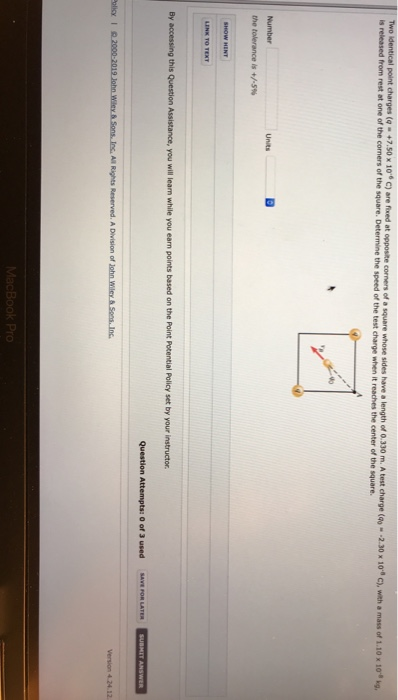at opposee comen oatquare whose sides have a length of 0.330 m. A test charge (ao "-2.30 x 10'a C), with a mass of 1.10 x 10-8 s released from rest at one of the corners of the square. Determine the speed of the test charge when it reaches the center of the square Number Units